## Convert Inches to Centimeters or Vice Versa in Excel Worksheets

by Avantix Learning Team | Updated October 14, 2022

Applies to: Microsoft® Excel® 2010, 2013, 2016, 2019, 2021 and 365 (Windows)

You can convert cm to inches (inches to centimeters or centimetres) using formulas with operators or functions in Excel. This is useful since the regional settings on a computer affect the default measurement system used in Excel and other programs.

Recommended articles: How to Hide Formulas from Clients, Colleagues and Other Users

Do you want to learn more about Excel? Check out our virtual classroom or live classroom Excel courses >

The following are the conversion amounts:

1 inch = 2.54 cm

1 cm = 0.3937 inch

### Divide to convert from cm to inches

To convert from cm to inches by dividing:

1. Click in a cell where you want to insert the converted amount.
2. Type =cell to convert/2.54 (for example =A2/2.54)
3. Press Enter.

In the following example, the conversion formula is entered in column B: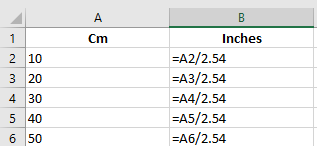### Multiply to convert from inches to cm

To convert from inches to cm by multiplying:

1. Click in a cell where you want to insert the converted amount.
2. Type =cell to convert*2.54 (for example =A2*2.54)
3. Press Enter.

In the following example, the conversion formula is entered in column B: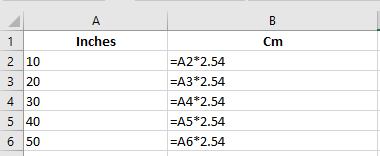### Use the CONVERT function

You can also use the CONVERT function to convert measurements.

The CONVERT function uses the following syntax: =CONVERT(Cell to convert,Units to convert from,Units to convert to).

The units must be entered in quotation marks or double quotes (such as "cm").

### Convert cm to inches using the CONVERT function

To convert cm to inches using the CONVERT function:

1. Click in a cell where you want to insert the converted amount.
2. Type =CONVERT(cell to convert,"cm","in"). For example, type =CONVERT(A2,"cm","in").
3. Press Enter.

In the following example, the conversion formula is entered in column B: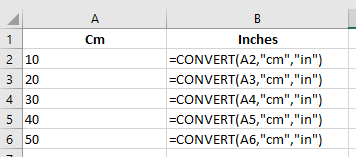### Convert inches to cm using the CONVERT function

To convert inches to cm using the CONVERT function:

1. Click in a cell where you want to insert the converted amount.
2. Type =CONVERT(cell to convert,"in","cm"). For example, type =CONVERT(A2,"in","cm").
3. Press Enter.

In the following example, the conversion formula is entered in column B: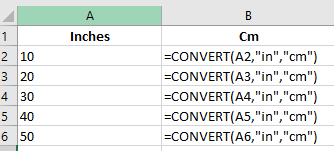There are also other units you can use with the CONVERT function.

This article was first published on November 27, 2020 and has been updated for clarity and content.

#### More resources

How to Highlight Errors, Blanks and Duplicates in Excel Worksheets

3 Excel Strikethrough Shortcuts to Cross Out Text or Values in Cells

How to Replace Blank Cells with a Value from the Cell Above in Excel

How to Use Flash Fill in Excel to Clean or Extract Data (Beginner's Guide)

#### Related courses

Microsoft Excel: Data Analysis with Functions, Dashboards and What-If Analysis Tools

Microsoft Excel: Introduction to Power Query to Get and Transform Data

Microsoft Excel: New and Essential Features and Functions in Excel 365

Microsoft Excel: Introduction to Visual Basic for Applications (VBA)

VIEW MORE COURSES >

Our instructor-led courses are delivered in virtual classroom format or at our downtown Toronto location at 18 King Street East, Suite 1400, Toronto, Ontario, Canada (some in-person classroom courses may also be delivered at an alternate downtown Toronto location). Contact us at info@avantixlearning.ca if you'd like to arrange custom instructor-led virtual classroom or onsite training on a date that's convenient for you.

## Excel XLOOKUP Function (Syntax + Formula Examples)

The XLOOKUP function is a replacement for Excel's traditional VLOOKUP function (as well as HLOOKUP and INDEX / MATCH functions). It has a new set of arguments and is available in Excel 2021 and 365. It allows you to look up a value from an array in a range or table and return one or more results. One of the primary benefits of XLOOKUP is that it can look up from columns to the left in a data set and return a range.

Microsoft, the Microsoft logo, Microsoft Office and related Microsoft applications and logos are registered trademarks of Microsoft Corporation in Canada, US and other countries. All other trademarks are the property of the registered owners.

Avantix Learning |18 King Street East, Suite 1400, Toronto, Ontario, Canada M5C 1C4 | Contact us at info@avantixlearning.ca

Summary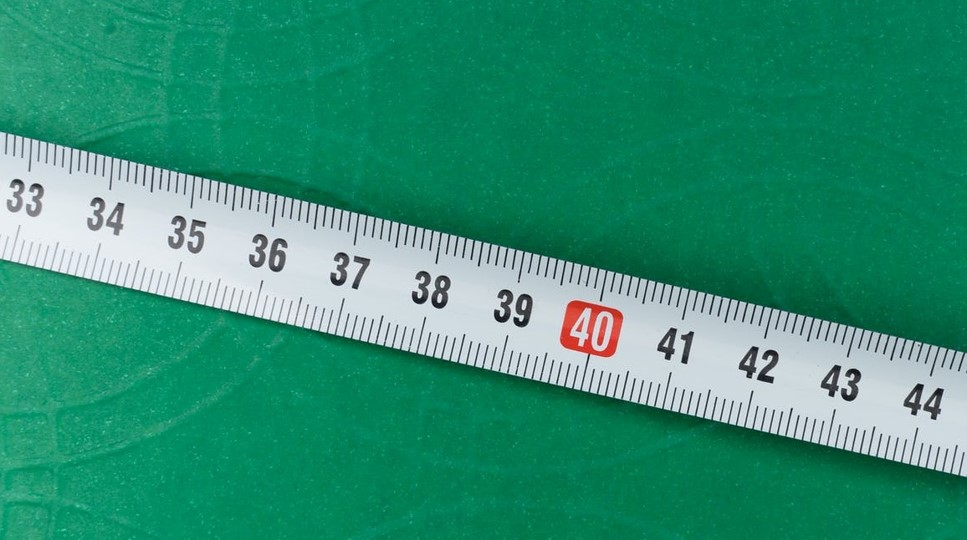Article Name
How to Convert Cm to Inches in Excel (or Inches to Cm)
Description
You can convert cm to inches (inches to centimeters or centimetres) using formulas with operators or functions in Excel. This is useful since the regional settings on a computer affect the default measurement system used in Excel and other programs.
Author
Publisher Name
Avantix Learning